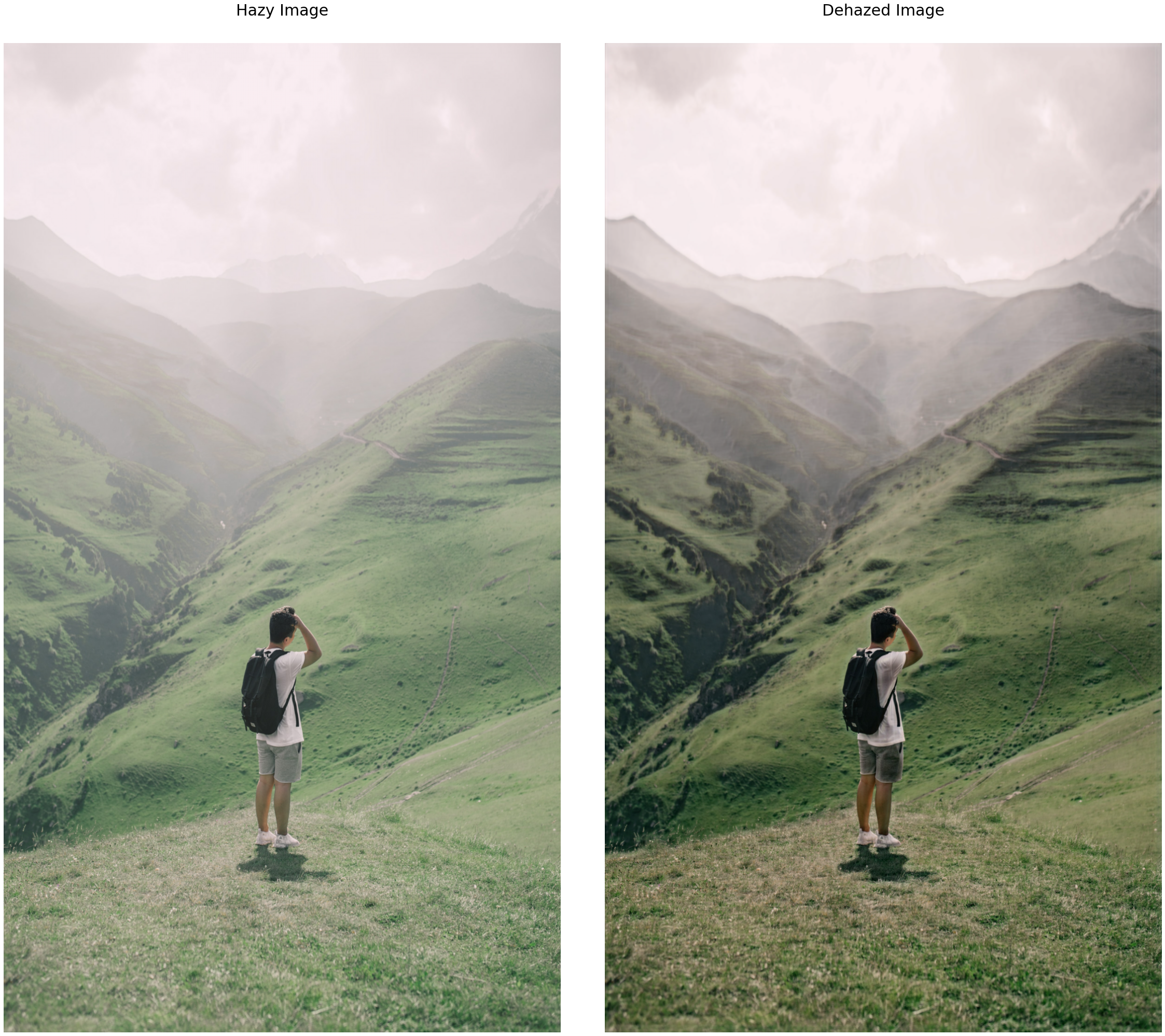# Generic Model-Agnostic CNN(GMAN) For Single Image Dehzing

Original article was published by Sanchit Vijay on Deep Learning on Medium## You can get the complete code from my GitHub.

This function takes the path to the image and read it and decode it into a uint8 tensor. Then we resize it to 412×548, image size in the dataset is 413×550 but the network function is creating issues because of odd input values. Finally, we normalize it and returns the image in the form of normalized tensors.

This function takes the path to the original and hazy image then make a split dictionary with keys: ‘train’, ‘val’ with 90% training data and 10% validation data. If you go through the dataset then the original images have a name like ‘0011’ and the corresponding hazy images have a name like ‘0011_0.85_0.2’, so every original image has more than one hazy image where numbers after underscore represent some kind of ratio in which haze is added to the original image. So below function, groups, the original image, and corresponding hazy images and returns them.

The below function applies the from_tensor_slices() function on the original and hazy image paths of the training set followed by a mapping function that loads the image(load_image). Then after that zip both the training datasets. Do the similar for the validation dataset. Finally return both datasets.

We use the below function to display the output of validation data after the training of the individual epoch. It takes a model, hazy, and original image as an argument.

## Network function

Below is the network function. We’ve used Conv2D, Conv2DTranspose to construct this function. First is the GMAN network in which all the layers have several filters as 64 except encoding layers having 128 and the final output layer has 3 channels(RGB). After GMAN, Parallel Network(PN) has dilated convolution layers with all layers having 64 filters except the last one with 3 channels(RGB). I’ve explained architecture in detail above.

## Training

The best thing I learned from this project is how to custom train a model. Usually fit, predict looks fascinating but the real way of training is this. The below function trains the model. In each epoch, we have a training loop and a validation loop. In the training loop, we take the training data and compute the gradients and apply them to calculate training loss. In the validation loop, we take those gradients computed in that epoch and apply them on validation data to check the output(using display_img function) and validation loss. Finally, we save the model(weights, variables, etc) of that epoch and reset the loss metrics.

Now before we train our model, we’ll define some hyperparameters. I’m using a batch size of 8 because above that GPU runs out of memory. We are not initializing kernel weights as zero, rather using random normal initialization is providing better results. And to reduce overfitting, L2 regularizer with weight decay of 1e-4 is used. Note that every layer is not having the same kernel initialization, it’s according to the research paper. Finally, call the training function.

## Evaluation(Testing)

Now I’ve taken some random foggy(naturally hazed) images from google and tested on them, the below function is used for that.

One of the test output is shown above and the others are below. You can check the rest here. You can check the validation output here.